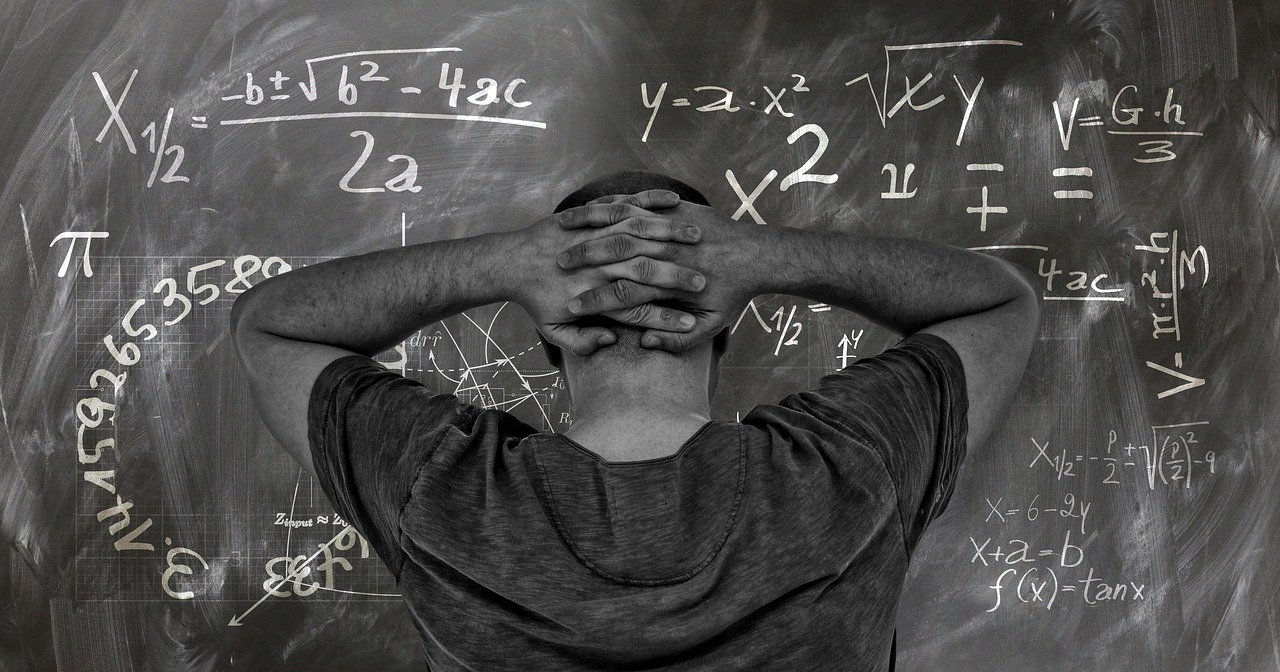How Useful is Mathematical Literacy for Grade 12 CAPS:

The subject Mathematical Literacy should enable the learner to become a self-managing person, a contributing worker and a participating citizen in a developing democracy. The teaching and learning of Mathematical Literacy
should thus provide opportunities to analyse problems and devise ways to work mathematically in solving such problems. Opportunities to engage mathematically in this way will also assist learners to become astute consumers of the mathematics reflected in the media.

## Mathematical Literacy for Grade 12, 11, and 10 Topics

There are five key elements of Mathematical Literacy.

## Mathematical Literacy involves the use of elementary mathematical content.

The mathematical content of Mathematical Literacy is limited to those elementary mathematical concepts and skills that are relevant to making sense of numerically and statistically based scenarios faced in the everyday lives of individuals (self-managing individuals) and the workplace (contributing workers), and to participating as critical citizens in social and political discussions. In general, the focus is not on abstract mathematical concepts.

As a rule of thumb, if the required calculations cannot be performed using a basic four-function calculator, then the calculation is in all likelihood not appropriate for Mathematical Literacy. Furthermore, since the focus in

Mathematical Literacy is on making sense of real-life contexts and scenarios, in the Mathematical Literacy classroom mathematical content
should not be taught in the absence of context.

## Mathematical Literacy involves authentic real-life contexts

In exploring and solving real-world problems, it is essential that the contexts learners are exposed to in this subject are authentic (i.e. are drawn from genuine and reaslitic situations) and relevant, and relate to daily life, the workplace and the wider social, political and global environments.

Wherever possible, learners must be able to work with actual real-life problems and resources, rather than with problems developed around constructed, semi-real, contrived and/ or fictitious scenarios. E.g. learners must be exposed to real accounts containing complex and “messy” figures rather than contrived and constructed replicas containing only clean and rounded figures.

Alongside using mathematical knowledge and skills to explore and solve problems related to authentic real-life contexts, learners should also be expected to draw on non-mathematical skills and considerations in making sense of those contexts. E.g. although calculations may reveal that a 10 kg bag of maize meal is the most cost-effective, consideration of the context may dictate that the 5 kg bag will have to be bought because the 10 kg bag cannot fit inside the taxi and/or the buyer does not have enough money to buy the 10 kg bag and/or the buyer has no use for 10 kg, etc.

In other words, mathematical content is simply one of many tools that learners must draw on in order to explore and make sense of appropriate contexts.

## More Mathematical Literacy Resources

View all Mathematical Literacy Resources

## Mathematical Literacy involves solving familiar and unfamiliar problems.

It is unrealistic to expect that in the teaching of Mathematical Literacy learners will always be exposed to contexts that are specifically relevant to their lives, and that they will be exposed to all of the contexts that they will one day encounter in the world. Rather, the purpose of this subject is to equip learners with the necessary knowledge and skills to be able to solve problems in any context that they may encounter in daily life and in the workplace, irrespective of whether the context is specifically relevant to their lives or whether the context is familiar.

Learners who are mathematically literate should have the capacity and confidence to interpret any real-life context that they encounter, and be able to identify and perform the techniques, calculations and/or other considerations needed to make sense of the context. In this sense Mathematical Literacy develops a general set of skills needed to deal with
a particular range of problems.

If Mathematical Literacy is seen in this way, then a primary aim in this subject is to equip learners with a set of skills that transcends both the mathematical content used in solving problems and the context in which the problem is situated. In other words, both the mathematical content and the context are simply tools: the mathematical content provides learners with a means through which to explore contexts; and the contexts add meaning to the mathematical content. But what is more important is that learners develop the ability to devise and apply both mathematical and
non-mathematical techniques and considerations in order to explore and make sense of any context, whether the
context is familiar or not.

My Courses has a large Questions and Answers repository for the most popular High School and Tertiary Schools subjects. This comes in handy when doing your revision or preparing for exams, tests, research tasks, and assignments.

Hey, Grade 12 Learners. My Courses portal has everything you needed to ace your matric exams, tests, assessments, research tasks, and assignments. Feel free to explore all resources for grade 12 learners, such as Study Guides, Department of Basic Education Past Exam Papers with Memos, and Speech Topics.